# NSCS 344, Week 2: Matlab Concepts

## Help and doc commands

This week we introduced a few built-in Matlab functions like mean, plot, set, and gca. One question I often get is how you can learn how to use these functions outside of the specific cases covered in the examples. There are two really helpful functions in Matlab you can use for this "help" and "doc" both of which you type directly into the Command Window.
Help gives you help in the Command Window itself. For example if you want help on a specific function (e.g. plot) then you just type the following, which tells you more than you will ever need to know about the plot function.
help plot
plot Linear plot. plot(X,Y) plots vector Y versus vector X. If X or Y is a matrix, then the vector is plotted versus the rows or columns of the matrix, whichever line up. If X is a scalar and Y is a vector, disconnected line objects are created and plotted as discrete points vertically at X. plot(Y) plots the columns of Y versus their index. If Y is complex, plot(Y) is equivalent to plot(real(Y),imag(Y)). In all other uses of plot, the imaginary part is ignored. Various line types, plot symbols and colors may be obtained with plot(X,Y,S) where S is a character string made from one element from any or all the following 3 columns: b blue . point - solid g green o circle : dotted r red x x-mark -. dashdot c cyan + plus -- dashed m magenta * star (none) no line y yellow s square k black d diamond w white v triangle (down) ^ triangle (up) < triangle (left) > triangle (right) p pentagram h hexagram For example, plot(X,Y,'c+:') plots a cyan dotted line with a plus at each data point; plot(X,Y,'bd') plots blue diamond at each data point but does not draw any line. plot(X1,Y1,S1,X2,Y2,S2,X3,Y3,S3,...) combines the plots defined by the (X,Y,S) triples, where the X's and Y's are vectors or matrices and the S's are strings. For example, plot(X,Y,'y-',X,Y,'go') plots the data twice, with a solid yellow line interpolating green circles at the data points. The plot command, if no color is specified, makes automatic use of the colors specified by the axes ColorOrder property. By default, plot cycles through the colors in the ColorOrder property. For monochrome systems, plot cycles over the axes LineStyleOrder property. Note that RGB colors in the ColorOrder property may differ from similarly-named colors in the (X,Y,S) triples. For example, the second axes ColorOrder property is medium green with RGB [0 .5 0], while plot(X,Y,'g') plots a green line with RGB [0 1 0]. If you do not specify a marker type, plot uses no marker. If you do not specify a line style, plot uses a solid line. plot(AX,...) plots into the axes with handle AX. plot returns a column vector of handles to lineseries objects, one handle per plotted line. The X,Y pairs, or X,Y,S triples, can be followed by parameter/value pairs to specify additional properties of the lines. For example, plot(X,Y,'LineWidth',2,'Color',[.6 0 0]) will create a plot with a dark red line width of 2 points. Example x = -pi:pi/10:pi; y = tan(sin(x)) - sin(tan(x)); plot(x,y,'--rs','LineWidth',2,... 'MarkerEdgeColor','k',... 'MarkerFaceColor','g',... 'MarkerSize',10) See also plottools, semilogx, semilogy, loglog, plotyy, plot3, grid, title, xlabel, ylabel, axis, axes, hold, legend, subplot, scatter. Documentation for plot Other functions named plot
Note that often the most useful stuff about the function is in the first few lines.
Doc works in a similar way, but pops up a separate and more modern-looking window that can have more information and some worked examples that can be really helpful
doc plot
Help and doc are really, really helpful. Practice looking up the documentation for another function (e.g. mean), click on the hyperlinks that appear in the doc and at the end of the help output. I have been coding in Matlab for more than 20 years (actually before the "doc" command even existed) and I use both of these functions on a regular basis.

## Functions

In addition to using Matlab's built-in functions, you can also make functions of your own. Once you've made them, they work just like Matlab's own functions. The recipe for making functions looks like this ..
• Create a new file in the Editor - either click on the "New Script" button in the toolbar or hit cmd+N (mac) or control+N (windows) in the Editor.
• Start the first line with the key word "function"
• Complete the first line with how you want to call your function. For example, if you want to make the function "my_function" and have it output a variable called "Z" based on input variables "X" and "Y" you would write
function Z = my_function(X, Y)
Note if you want to have more than one ouput (Z1, and Z2), then you need to use square brackets around the two outputs like this
function [Z1, Z2] = myfunction(X, Y)
• This is a good point to save your function. If you do it now, after you've written the "function" line, Matlab will automatically name the file with the same name as your function (so "myfunction" in this case).
*** IMPORTANT *** Make sure your function file is in the same directory as the script you are going to be calling it from. If they are in different directories, Matlab will not be able to "see" your function when you try to call it from your script. (There are other ways around this by setting Matlab's paths, but this is a good solution for this class - and it also means that all of your code will be in the same directory so we can grade it!)
• Then you write your code for the function inside the function. Make sure that you use all the inputs you give it and also make sure that you actually create the variables you said you wanted as outputs (Z in our first example, Z1 and Z2 in our second example).
For example, this would be a perfectly good example of a function ...
% This is an example of a good function
function [Z1, Z2] = myfunction(X, Y)
Z1 = X * Y;
Z2 = Y - X;
However, this next example would give an error if you tried to run it because you haven't defined Z2 - instead you called Y - X Z3.
% This is an example of a bad function that will give an error
function [Z1, Z2] = myfunction(X, Y)
Z1 = X * Y;
Z3 = Y - X;
Another example of something going wrong with a function (I see this bug a lot, and it occurs often if you are copy-pasting code from a script) is if you redefine your inputs inside the code. For example,
% This is an example of a bad function that will _not_ give an
% error, but will not run as expected. Regardless of what
% values you give it for X and Y, they will always be set to
% 10 and 5 _inside_ the function
function [Z1, Z2] = myfunction(X, Y)
X = 10;
Y = 5;
Z1 = X * Y;
Z2 = Y - X;
• Finally, to call your function you need to add a line to your script - and save your script in the same folder that your function is in. For example, in your script, to call the function with X = 3 and Y = 6, you could write the following, which will create two variables (Z1 and Z2) that you can see in your Workspace
[Z1, Z2] = myfunction(3, 6);
Note: The only variables you can get out of your function are the variables you say are outputs in the first line. For example, if your function reads
% This is an example of a good function
function [Z1, Z2] = myfunction(X, Y)
Z1 = X * Y;
Z2 = Y - X;
newVariable = X / Y;
You will not be able to get "newVariable" into your script unless you add a third output to your function like this ...
% This is an example of a good function
function [Z1, Z2, newVariable] = myfunction(X, Y)
Z1 = X * Y;
Z2 = Y - X;
newVariable = X / Y;
and call it in your script like this ...
[Z1, Z2, newVariable] = myfunction(X, Y);

## Vectors and Matrices

Sometimes we don't just want to deal with one number, instead we want to deal with a whole bunch of numbers at once. One example is if we want to track and store the value of some variable for many time points, in this case we would need one value per time point. Another would be if we have a set of data that involves multiple conditions - for example viewing time and coherence for the dots. Here we have one data point (the accuracy) for each combination of viewing times and dot coherence. Vectors and Matrices are Matlab's way of letting us do this.

## Vectors

A vector lets us store a list of numbers (e.g. the numbers 1, 9, 10, 15) and then refer to those numbers later (e.g. the third number in the list is 10). In Matlab notation we can create a vector using the square brackets like this ...
myList = [1 9 10 15]
myList = 1×4
1 9 10 15
And then I can refer to individual elements of the list like this ...
myList(3)
ans = 10
I can also reset a given element. For example, if I want to change the 3rd element to 100 I could write
myList(3) = 100
myList = 1×4
1 9 100 15
I can also perform arithmetic on my vector. For example, if I want to multiply all elements by 100 and assign that to a new variable
newList = 100 * myList
newList = 1×4
100 900 10000 1500
Finally, I can get the number of elements in my vector, the length of the list using the length command
length(myList)
ans = 4
This is especially useful if I want to loop through all the elements in my list ...
for i = 1:length(myList)
myList(i)
end
ans = 1
ans = 9
ans = 100
ans = 15

## Matrices

Matrices are the generalization of vectors to more than one dimension. Vectors are one-dimensional in that they are a list of numbers effectively on a line. Matrices are numbers in a table. We can also assign matrices using the square brackets. For example, like this
myMatrix = [
1 2 3
4 5 6
]
myMatrix = 2×3
1 2 3 4 5 6
This gives us a 2 x 3 matrix, which is a table of values.
I can look up any particular element in the matrix using an index for the row and then the column. For example, if I want the data point in row 2 column 1 of myMatrix I can write
myMatrix(2, 1)
ans = 4
I can also refer to a whole row or column of a matrix using a colon. For example if I want the whole of the second row I can write
myMatrix(2,:)
ans = 1×3
4 5 6
And if I want the whole of the third column I can write
myMatrix(:,3)
ans = 2×1
3 6
Apart from being multidimensional, matrices share many of the properties of vectors. So I can perform artimetic on them like this ...
newMatrix = myMatrix * 100
newMatrix = 2×3
100 200 300 400 500 600
I can also change the value of just one element
myMatrix(2,1) = 100
myMatrix = 2×3
1 2 3 100 5 6
The length function does work, but it gives us the length of the longest dimension (in this case 3)
length(myMatrix)
ans = 3
But that's no good for looping over all elements because I need the number of elements in each dimension of myMatrix. To do this, I need to use the size function
size(myMatrix)
ans = 1×2
2 3
This gives a vector output of the sizes of the two dimensions of myMatrix. If I only want the number of elements in one dimensions, I can simply write
size(myMatrix, 1)
ans = 2
To get the number of elements in the first dimension or
size(myMatrix, 2)
ans = 3
To get the number of elements in the second dimension. This allows me to loop over all elements in my matrix using two for loops
for i = 1:size(myMatrix , 1)
for j = 1:size(myMatrix, 2)
myMatrix(i,j)
end
end
ans = 1
ans = 2
ans = 3
ans = 100
ans = 5
ans = 6

## Matrices with more than two dimensions

It's possible to make Matrices with more than two dimensions. Mathematically these are called tensors. It's harder to write these using square brackets, but easy to define them using loops
for i = 1:4
for j = 1:3
for k = 1:2
myTensor(i,j,k) = (i-1)*3*3 + (j-1)*2 + k;
end
end
end
myTensor
myTensor =
myTensor(:,:,1) = 1 3 5 10 12 14 19 21 23 28 30 32 myTensor(:,:,2) = 2 4 6 11 13 15 20 22 24 29 31 33
Now I can refer to a whole matrix within my tensor like this ...
myTensor(:, :, 1)
ans = 4×3
1 3 5 10 12 14 19 21 23 28 30 32
or like this
myTensor(1,:,:)
ans =
ans(:,:,1) = 1 3 5 ans(:,:,2) = 2 4 6
Or like this
myTensor(:,2, :)
ans =
ans(:,:,1) = 3 12 21 30 ans(:,:,2) = 4 13 22 31
I can also refer just to a vector like this
myTensor(:,1,1)
ans = 4×1
1 10 19 28
etc ...

## Basic plots

Often, when we've stored some data in a vector or matrix we'd like to plot it. The simplest way to do this is with the "plot" command. If I use the plot command on a vector it simply plots the values of the vector on the y-axis against the element number of the x-axis. For example:
% define a vector to plot
myVector = [1 2 3 9 8 7];
% plot the vector
plot(myVector)
If I would like something other than the element number on the x-axis, I need to create another vector to give me the list of values I need for the corresponding x locations. For example, if I want to reverse the plot I could make the x values decrease ...
xData = [6 5 4 3 2 1];
plot(xData, myVector)
If my data are in a two-dimensional matrix, then plotting the matrix will give more than one line, one for each column of data
myMatrix = [
1 9
2 8
3 7
4 6
5 5
6 4
];
plot(myMatrix)
Matlab automatically changes the colors.
If I'd like to plot something else on my plot I need to use the "hold on" command. For example:
plot(myVector)
hold on
plot(myMatrix)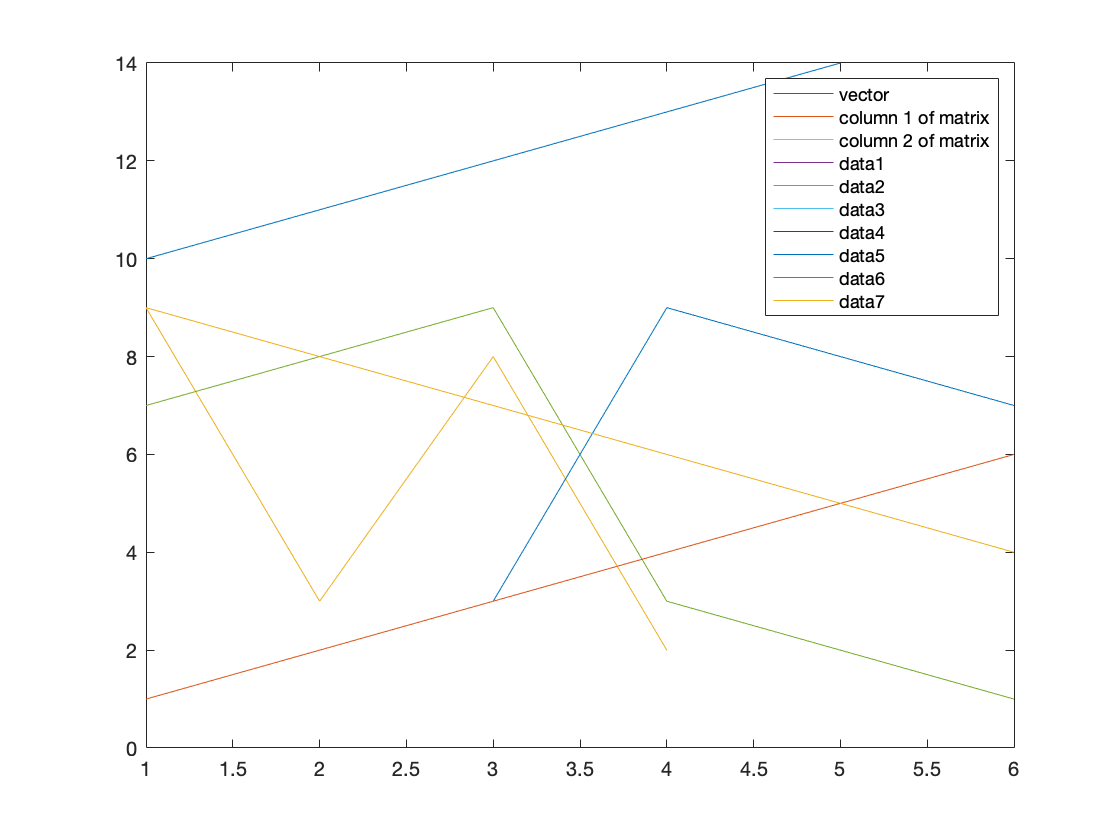This plots three lines - one for myVector (the blue line) and two for the two columns of myMatrix (the red and yellow lines). Note there's some overlap between the blue and red lines.
If I want to clear my figure to plot something new I can use the clf command (for clear figure)
clf
plot([1 2 3])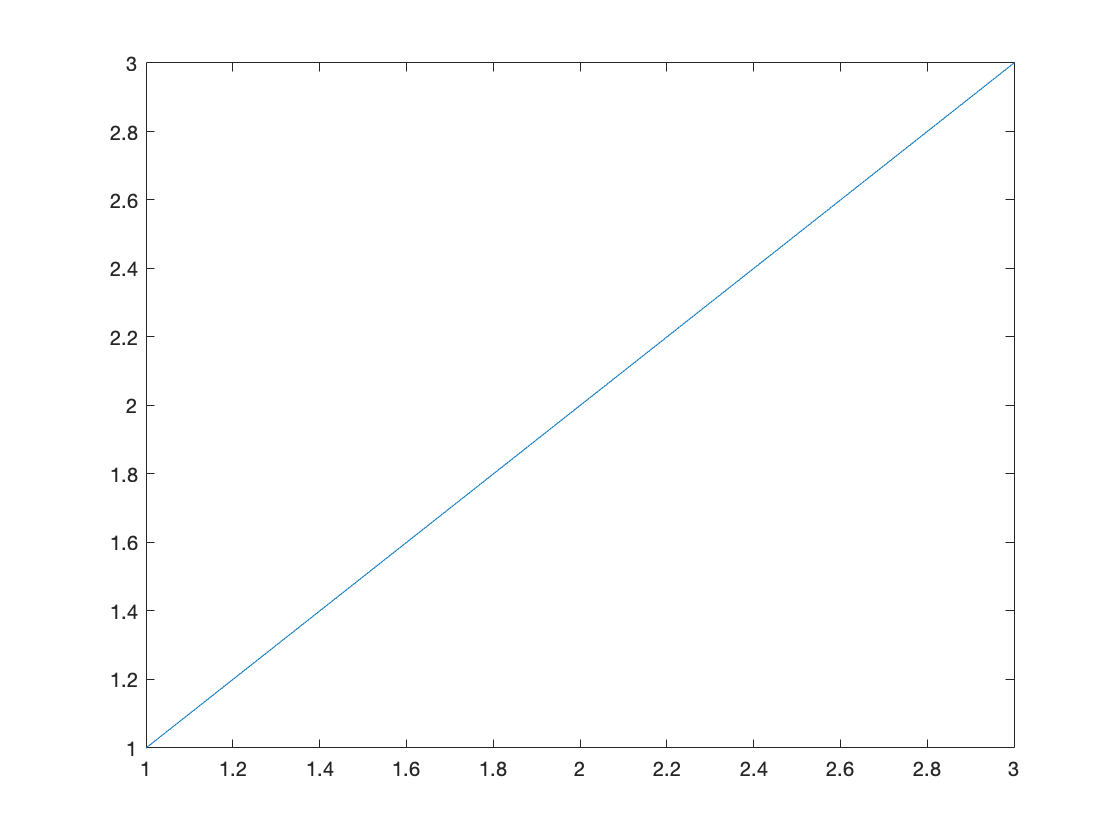## Better looking plots

The Matlab default plots look pretty ugly and they are also missing a bunch of information. For example, we'd like to add labels to the axes, a legend if there's more than one line, and maybe a title. We'd also like to change the colors and maybe some other properties of the lines. These can all be achieved using a few Matlab commands ...
First labels can be added to the x and y axis using xlabel and ylabel
clf;
plot([1 2 3 4 5])
xlabel('this is an x label')
ylabel('this is a y label')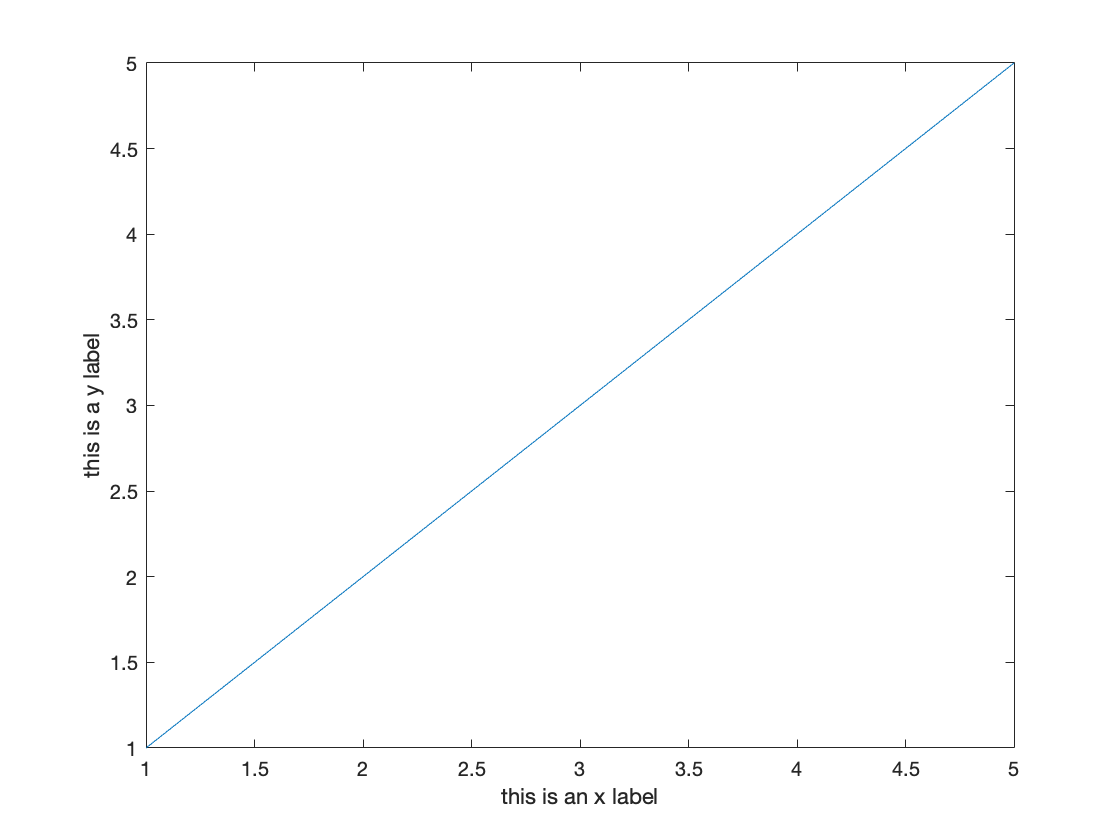Titles can be added using the title command
clf;
plot([1 2 3 4 5])
xlabel('this is an x label')
ylabel('this is a y label')
title('this is a title')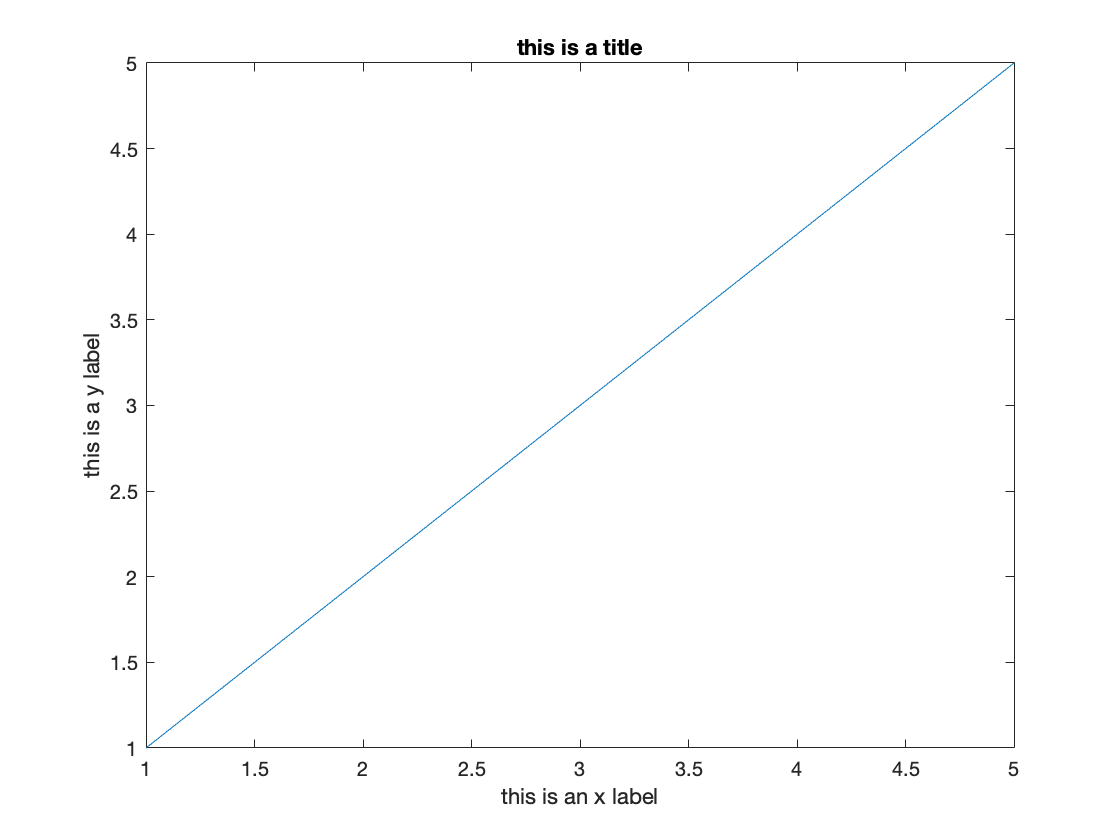If I want to increase my font size I can use the "set" command like this ...
clf;
plot([1 2 3 4 5])
xlabel('this is an x label')
ylabel('this is a y label')
title('this is a title')
set(gca, 'fontsize', 18)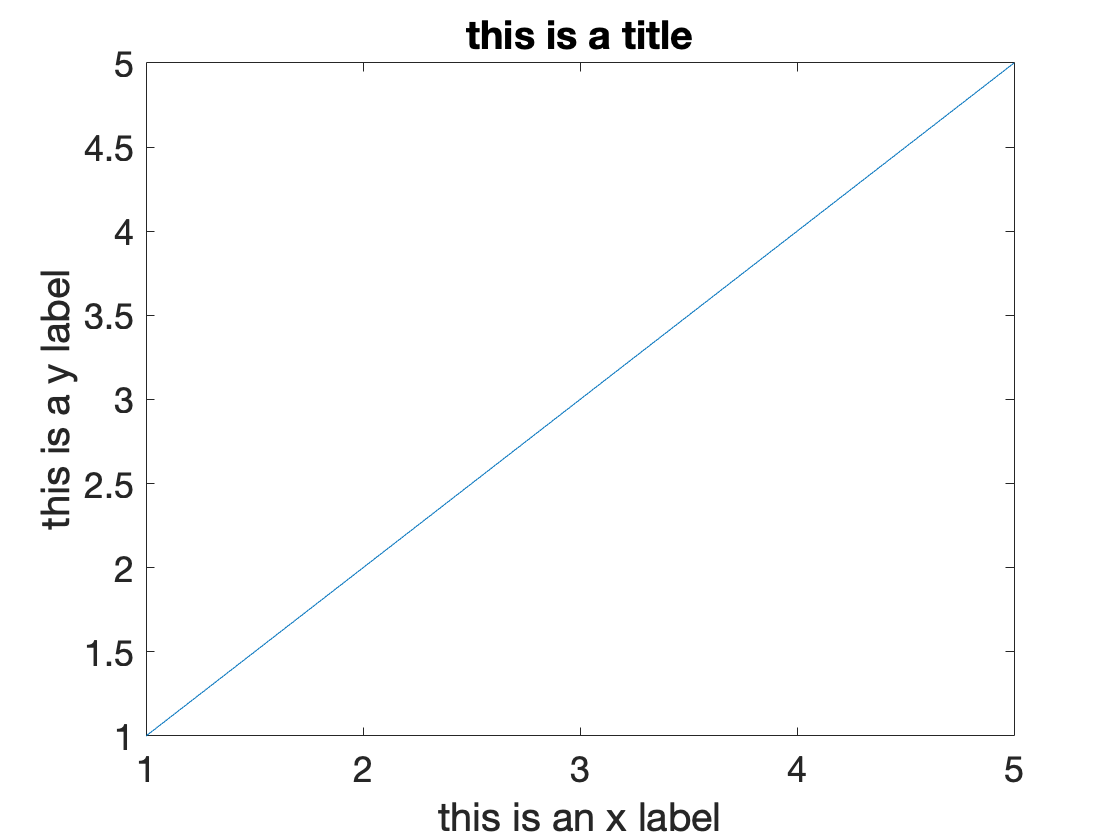The set command is a very general function, what it's doing here is changing the fontsize of the current axes (which it gets using the gca command - for get current axes). You can set all kinds of axis properties using the set command. To get a list of these properties you can just write
set(gca)
ALim: {} ALimMode: {'auto' 'manual'} AlphaScale: {'linear' 'log'} Alphamap: {} AmbientLightColor: {1×0 cell} Box: {[on] [off]} BoxStyle: {'full' 'back'} BusyAction: {'queue' 'cancel'} ButtonDownFcn: {} CLim: {} CLimMode: {'auto' 'manual'} CameraPosition: {} CameraPositionMode: {'auto' 'manual'} CameraTarget: {} CameraTargetMode: {'auto' 'manual'} CameraUpVector: {} CameraUpVectorMode: {'auto' 'manual'} CameraViewAngle: {} CameraViewAngleMode: {'auto' 'manual'} Children: {} Clipping: {[on] [off]} ClippingStyle: {'rectangle' '3dbox'} Color: {1×0 cell} ColorOrder: {} ColorOrderIndex: {} ColorScale: {'linear' 'log'} Colormap: {} ContextMenu: {} CreateFcn: {} DataAspectRatio: {} DataAspectRatioMode: {'auto' 'manual'} DeleteFcn: {} FontAngle: {'normal' 'italic'} FontName: {} FontSize: {} FontSizeMode: {'auto' 'manual'} FontSmoothing: {[on] [off]} FontUnits: {1×5 cell} FontWeight: {'normal' 'bold'} GridAlpha: {} GridAlphaMode: {'auto' 'manual'} GridColor: {1×0 cell} GridColorMode: {'auto' 'manual'} GridLineStyle: {1×5 cell} HandleVisibility: {'on' 'callback' 'off'} HitTest: {[on] [off]} InnerPosition: {} Interactions: {} Interruptible: {[on] [off]} LabelFontSizeMultiplier: {} Layer: {'bottom' 'top'} Layout: {} LineStyleOrder: {} LineStyleOrderIndex: {} LineWidth: {} MinorGridAlpha: {} MinorGridAlphaMode: {'auto' 'manual'} MinorGridColor: {1×0 cell} MinorGridColorMode: {'auto' 'manual'} MinorGridLineStyle: {1×5 cell} NextPlot: {1×4 cell} OuterPosition: {} Parent: {} PickableParts: {1×3 cell} PlotBoxAspectRatio: {} PlotBoxAspectRatioMode: {'auto' 'manual'} Position: {} PositionConstraint: {1×2 cell} Projection: {1×2 cell} Selected: {[on] [off]} SelectionHighlight: {[on] [off]} SortMethod: {'depth' 'childorder'} Tag: {} TickDir: {'in' 'out' 'both'} TickDirMode: {'auto' 'manual'} TickLabelInterpreter: {'none' 'tex' 'latex'} TickLength: {} Title: {} TitleFontSizeMultiplier: {} TitleFontWeight: {'normal' 'bold'} Toolbar: {} Units: {1×6 cell} UserData: {} View: {} Visible: {[on] [off]} XAxis: {} XAxisLocation: {1×3 cell} XColor: {1×0 cell} XColorMode: {'auto' 'manual'} XDir: {'normal' 'reverse'} XGrid: {[on] [off]} XLabel: {} XLim: {} XLimMode: {'auto' 'manual'} XMinorGrid: {[on] [off]} XMinorTick: {[on] [off]} XScale: {'linear' 'log'} XTick: {} XTickLabel: {} XTickLabelMode: {'auto' 'manual'} XTickLabelRotation: {} XTickMode: {'auto' 'manual'} YAxisLocation: {1×3 cell} YColor: {1×0 cell} YColorMode: {'auto' 'manual'} YDir: {'normal' 'reverse'} YGrid: {[on] [off]} YLabel: {} YLim: {} YLimMode: {'auto' 'manual'} YMinorGrid: {[on] [off]} YMinorTick: {[on] [off]} YScale: {'linear' 'log'} YTick: {} YTickLabel: {} YTickLabelMode: {'auto' 'manual'} YTickLabelRotation: {} YTickMode: {'auto' 'manual'} ZAxis: {} ZColor: {1×0 cell} ZColorMode: {'auto' 'manual'} ZDir: {'normal' 'reverse'} ZGrid: {[on] [off]} ZLabel: {} ZLim: {} ZLimMode: {'auto' 'manual'} ZMinorGrid: {[on] [off]} ZMinorTick: {[on] [off]} ZScale: {'linear' 'log'} ZTick: {} ZTickLabel: {} ZTickLabelMode: {'auto' 'manual'} ZTickLabelRotation: {} ZTickMode: {'auto' 'manual'}
One nice thing to change is the positions of the x and y ticks ...
clf;
plot([1 2 3 4 5])
xlabel('this is an x label')
ylabel('this is a y label')
title('this is a title')
set(gca, 'fontsize', 18, 'xtick', [1 3 5], 'ytick', 1:5)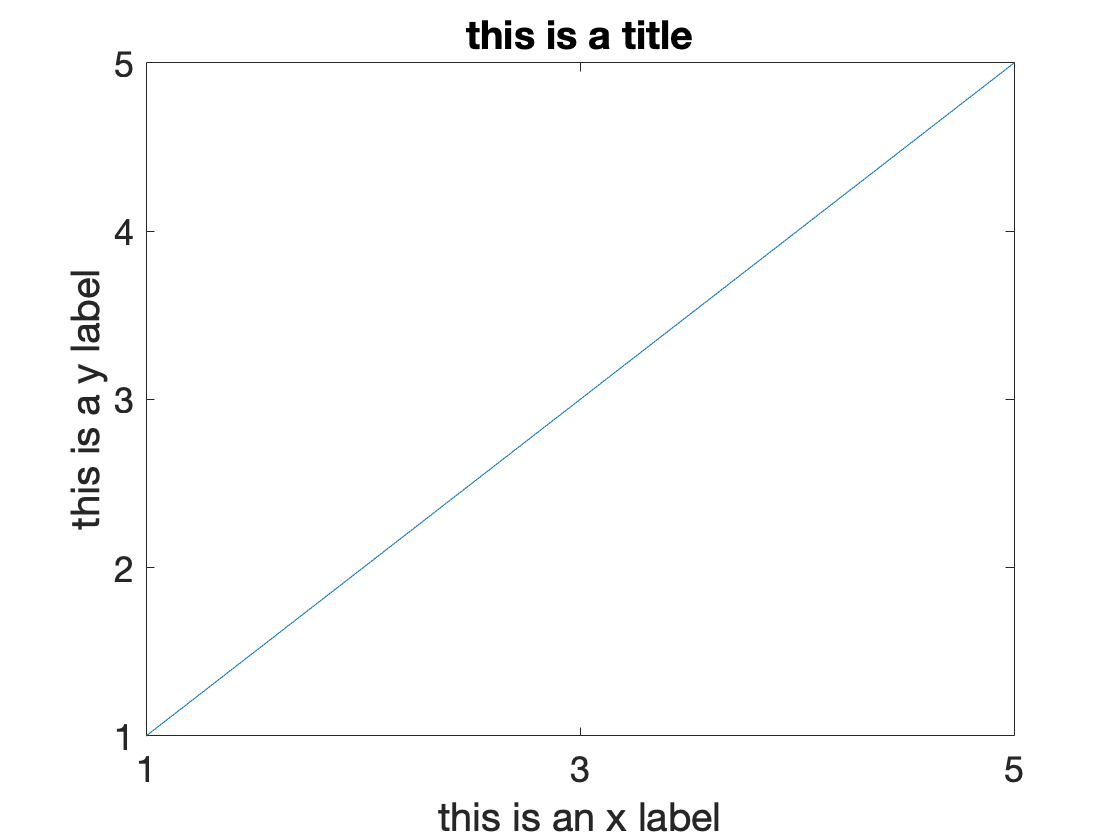If I want to change the thickness of the line I can also use the set command, but this time pass it a variable corresponding to the line itself. This I get by assigning an output variable for plot - like this ...
l = plot(1:20);
set(l, 'linewidth', 5)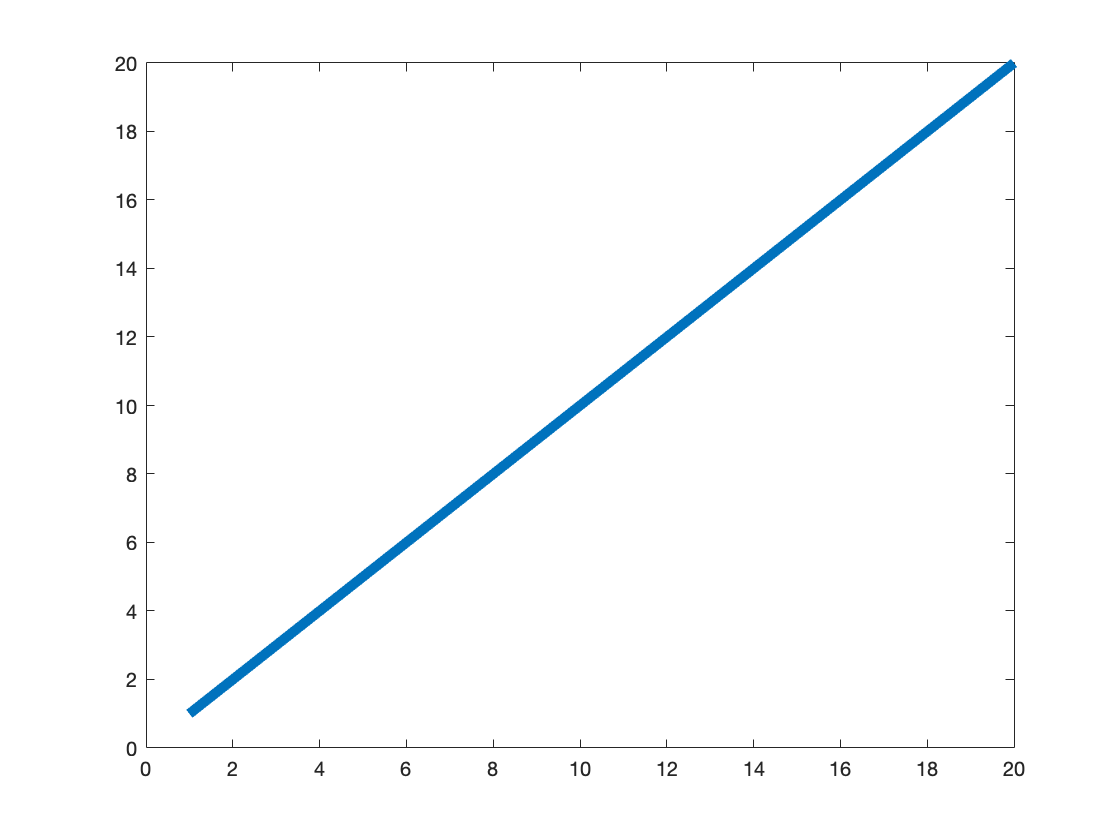I can also change the color of the line like this ...
l = plot(1:20);
AZ_bloom = [239 64 86]/256;
set(l, 'linewidth', 5, 'color', AZ_bloom)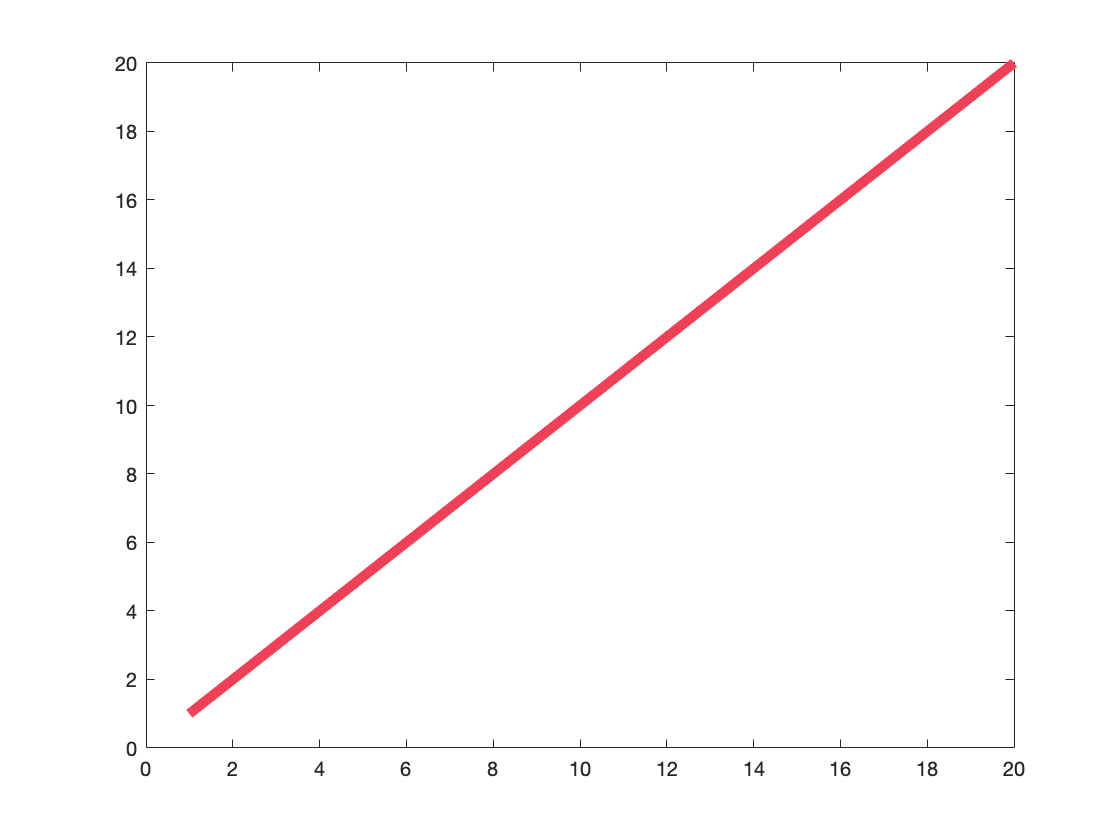Finally, I can add a legend (when I have multiple lines) like this ...
clf;
plot(10:13)
hold on
plot([
1 9
2 3
3 8
9 2])
legend({'vector' 'column 1 of matrix' 'column 2 of matrix'})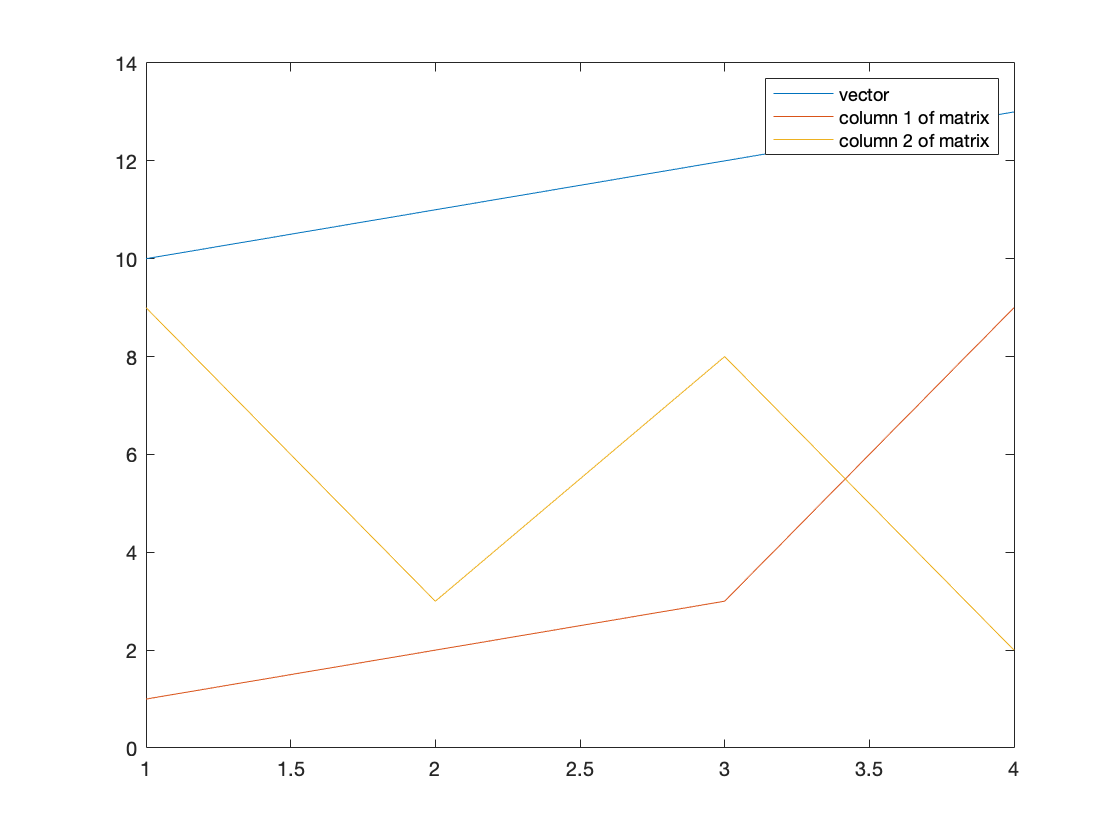To load and save data we (perhaps not surprisingly) use the load and save commands. Just typing "save" will save all the variables that are currently in the workspace to a file called matlab.mat file in the current directory
save
Saving to: /Users/bob/Dropbox/NSCS344_BOB/Week_01/matlab.mat
If I'd like a better name, I can specify that after the save command
save myFile
If I don't want to save in the current directory I can specify a path like this (to save it to the Desktop)
save ~/Desktop/myFile
And if I don't want to save all the variables, but just a few, I can list them afterwards like this
save myFile myVector myMatrix
Load works similarly. If I just type load it will load the matlab.mat file from the current directory